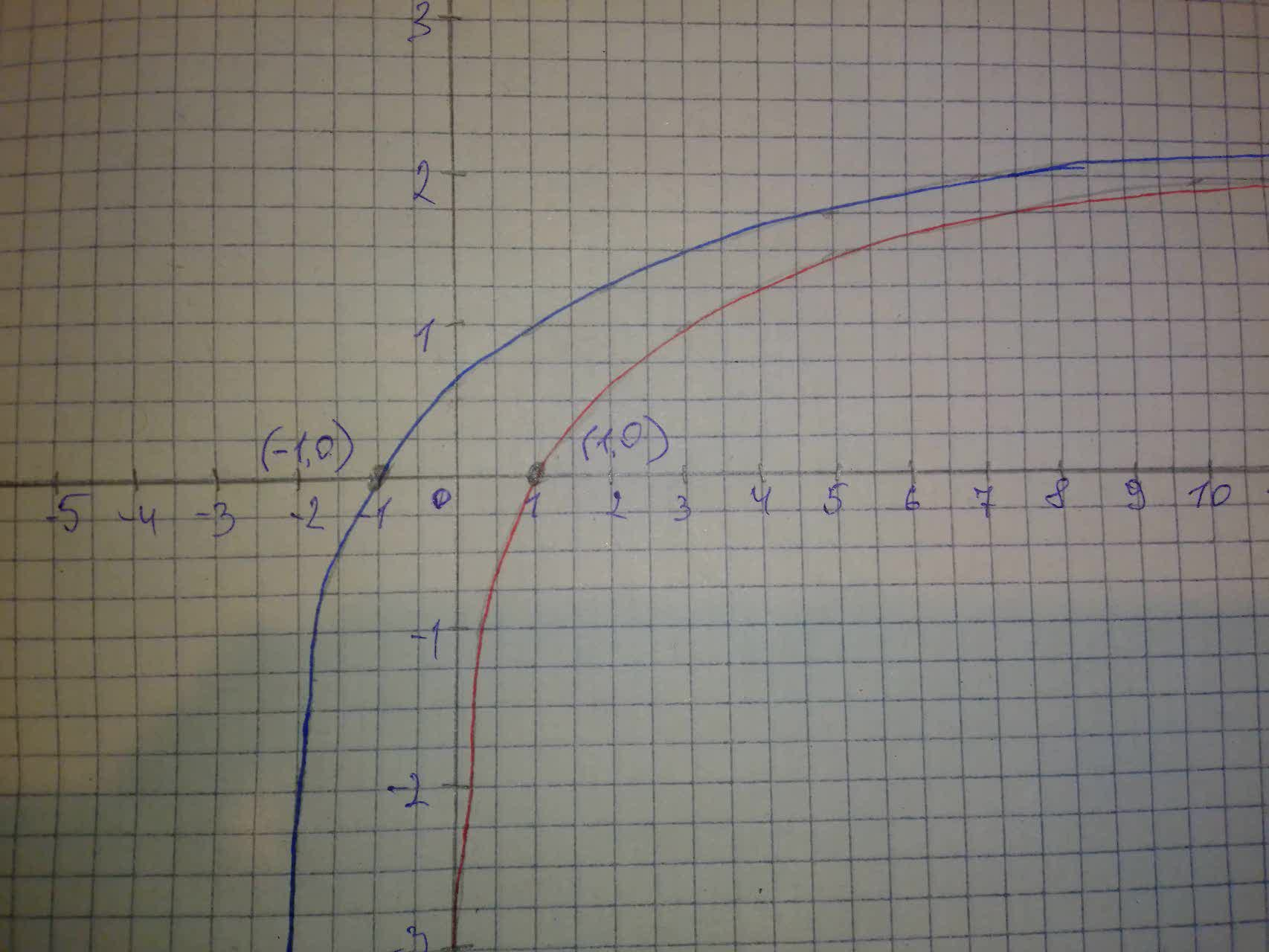# For y=\log_{3}(x+2) a. Use transformations of the graphs of y=\log_{2}xaflacatn 2021-08-15 Answered
For $y={\mathrm{log}}_{3}\left(x+2\right)$ a. Use transformations of the graphs of $y={\mathrm{log}}_{2}x$ and $y={\mathrm{log}}_{3}x$ to graph the given functions. b. Write the domain and range in interval notation. c. Write an equation of the asymptote.
You can still ask an expert for help

• Questions are typically answered in as fast as 30 minutes

Solve your problem for the price of one coffee

• Math expert for every subject
• Pay only if we can solve itsaiyansruleA
Step 1
a) Stert from the graph of the parent function $f\left(x\right)={\mathrm{log}}_{3}x$.
As we can see the given function $y={\mathrm{log}}_{3}\left(x+2\right)$ can be expressed in terms of the parent function f as $y=f\left(x+2\right)$
This indicates that the graph of the function $y={\mathrm{log}}_{3}\left(x+2\right)$ will be the same as the graph of the parent function $f\left(x\right)={\mathrm{log}}_{3}x$ shifted 2 units left.
See the graph in the picture below:Step 2
b) The domain of the function $y={\mathrm{log}}_{3}\left(x+2\right)$ is the interval: $\left(-2,+\mathrm{\infty }\right)$
The range of the function $y={\mathrm{log}}_{3}\left(x+2\right)$ is the interval $\left(-\mathrm{\infty },+\mathrm{\infty }\right)$
c) The vertical asymptote of the graph of this function is the line $x=-2$